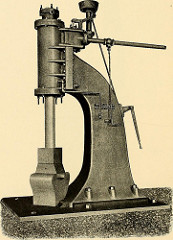#MTBF is Just the Mean, Right?

A conversation the other day involved how or why someone would use the mean of a set of data described by a Weibull distribution.

The Weibull distribution is great at describing a dataset that has a decreasing or increasing hazard rate over time. Using the distribution we also do not need to determine the MTBF (which is not all that useful, of course).

Walking up the stairs today, I wondered if the arithmetic mean of the time to failure data, commonly used to estimate MTBF, is the same as the mean of the Weibull distribution. Doesn’t everyone think about such things?

Doesn’t everyone think about such things? So, I thought, I’d check. Set up some data with an increasing failure rate, and calculate the arithmetic mean and the Weibull distribution mean.

## The Data Set

I opened R and using the random number generating function, rweibull, created 50 data points from a Weibull distribution with a shape (β) of 7 and scale (η) of 1,000.

Here’s a histogram of the data.

## Calculating the Mean Two Ways

Let’s say the randomly generated data is complete. No censoring, no replacements, etc. All fifty items ran for some amount of time and then failed. We could calculate the MTBF by tallying up all the time to failure data and dividing by the number of failures.

This is the arithmetic mean, that one we use commonly for all sorts of data summarization work.

Doing so we find the mean is 951.1.

Now is the mean of the Weibull distribution the same or not?

The formula for the mean of a Weibull distribution is$\displaystyle \bar{T}=\eta \centerdot \Gamma \left( \frac{1}{\beta }+1 \right)$

according to Reliawiki’s discussion of the Weibull Distribution.

Ok, let’s calculate the Weibull mean given the distribution has a β of 7 and η of 1,000. We find the Weibull mean as 935.4.

## Comparison and an Aha! Moment

Since 935.4 ≠ 951.1, I will conclude the two ways to calculate the mean is not the same. Hum, wait a minute. A set of random values from a distribution does not mean the data is best described by the generating distribution, especially for a small dataset.

So, let’s check something. If I generate 50,000 data points from the same distribution as above, the data should be very close to the distribution used to create the data.

With 50,000 data points, the arithmetic mean is 935.0, which is very close to the Weibull mean, 935.4, based on the β and η of the random generating function.

I have to now conclude the mean calculated both ways is same. Both determine the 1st moment of the dataset, the center of mass, etc.

My initial error was not determining the distribution parameters based on the data.

## Summary

Question answered. The calculation of MTBF or the mean from the data or based on the distribution parameters is the same.

That leaves the question of why anyone would want to calculate the mean of a set of time to failure data in the first place. We’ve been trying to convince you and everyone else to not bother doing so.

If you have a good reason to calculate the mean of a dataset with a clear increasing hazard rate, leave a comment below. Need to check my assumpiton that the Weibull mean is not all that useful and not worth the effort to calcualte using any method.I am an experienced reliability engineering and management consultant with my firm FMS Reliability. My passion is working with teams to create cost-effective reliability programs that solve problems, create durable and reliable products, increase customer satisfaction, and reduce warranty costs.

## 2 thoughts on “MTBF and Mean of Wearout Data”

1.WILLIAM THORLAY says:

Fred, in this example you said that there were 50 failures in 1200 hours. From zero to 600h, there were no failures. How did you come up with MTBF of 951.1?

1.Fred Schenkelberg says:

I knew I should have kept the raw data. What I did was generate 50 random number from a Weibull beta 7 eta 1,000 distribution. As the histogram shows an obvious increasing failure rate over time (not counting the last bin…)

The MTBF or mean is just the straight calculator mean, adding all the value and dividing by the number of items. The values ranged from about 600 to 1,200, yet the center of mass for the 50 values was at 951. Since there were only 50 random numbers generated it likely would fit a distribution near beta 7 eta 1,000, yet not exactly.

The Weibull derived mean is using the beta and eta withe Gamma function in the formula to calculate the mean – I suspected they should match, yet had never tried it.

Cheers,

Fred